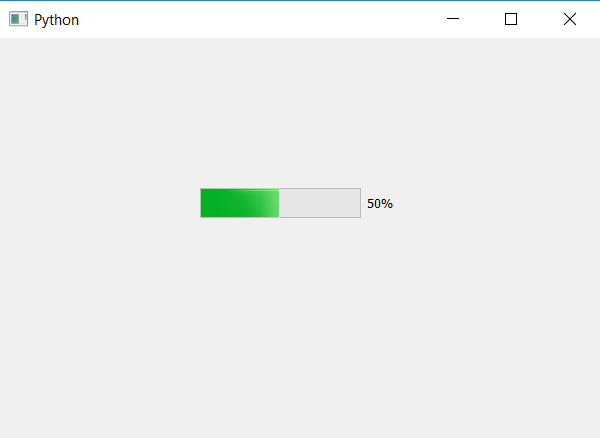Open in App
Not now

# PyQt5 | How to set value of Progress Bar ?

• Last Updated : 22 Apr, 2020

In this article we will see how to set the value of progress bar. Progress bar is basically a bar which is used to visualize the progression of an extended computer operation, such as a download, file transfer, or installation.

In order to set value to progress bar we will use `setValue` method.

Syntax : bar.setValue(value)

Argument : It takes integer as argument, which should vary from 0 to 100.

Action performed : It will set value to progress bar.

Code :

 `# importing libraries``from` `PyQt5.QtWidgets ``import` `*` `from` `PyQt5.QtGui ``import` `*` `from` `PyQt5.QtCore ``import` `*` `import` `sys`` ` ` ` `class` `Window(QMainWindow):``    ``def` `__init__(``self``):``        ``super``().__init__()`` ` `        ``# setting title``        ``self``.setWindowTitle(``"Python "``)`` ` `        ``# setting geometry``        ``self``.setGeometry(``100``, ``100``, ``600``, ``400``)`` ` `        ``# calling method``        ``self``.UiComponents()`` ` `        ``# showing all the widgets``        ``self``.show()`` ` `    ``# method for widgets``    ``def` `UiComponents(``self``):`` ` `        ``# creating progress bar``        ``bar ``=` `QProgressBar(``self``)`` ` `        ``# setting geometry to progress bar``        ``bar.setGeometry(``200``, ``150``, ``200``, ``30``)``         ` `        ``# setting value to progress bar``        ``bar.setValue(``50``)`` ` ` ` `# create pyqt5 app``App ``=` `QApplication(sys.argv)`` ` `# create the instance of our Window``window ``=` `Window()`` ` `# start the app``sys.exit(App.``exec``())`

Output :My Personal Notes arrow_drop_up